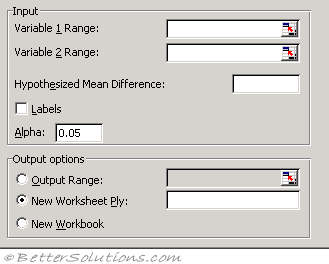# T-Test Paired Two Sample

T-Test is a special case of a one-way ANOVAVariable 1 Range - Enter the cell reference for the first range of data you want to analyze. The range must consist of a single column or row and contain the same number of data points as the second range.
Variable 2 Range - Enter the cell reference for the second range of data you want to analyze. The range must consist of a single column or row and contain the same number of data points as the first range.
Hypothesized Mean Difference - Enter the number you want for the shift in sample means. A value of 0 (zero) indicates that the sample means are hypothesized to be equal.
Labels - Select if the first row or column of your input ranges contains labels. Clear this check box if your input ranges have no labels; Microsoft Excel generates appropriate data labels for the output table.
Alpha - Enter the confidence level for the test. This value must be in the range 0...1. The alpha level is a significance level related to the probability of having a type I error (rejecting a true hypothesis).
Output Range - Enter the reference for the upper-left cell of the output table. Excel automatically determines the size of the output area and displays a message if the output table will replace existing data.
New Worksheet Ply - Click to insert a new worksheet in the current workbook and paste the results starting at cell A1 of the new worksheet. To name the new worksheet, type a name in the box.
New Workbook - Click to create a new workbook and paste the results on a new worksheet in the new workbook.

The t-Test analysis tools test the means of different types of populations.
t-Test: Two-Sample Assuming Equal Variances This analysis tool performs a two-sample student's t-test. This t-test form assumes that the means of both data sets are equal; it is referred to as a homoscedastic t-test. You can use t-tests to determine whether two sample means are equal.

t-Test: Paired Two Sample For Means This analysis tool and its formula perform a paired two-sample student's t-test to determine whether a sample's means are distinct. This t-test form does not assume that the variances of both populations are equal. You can use a paired test when there is a natural pairing of observations in the samples, such as when a sample group is tested twice, before and after an experiment. Note Among the results generated by this tool is pooled variance, an accumulated measure of the spread of data about the mean, derived from the following formula.